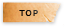# 生物與醫護統計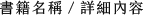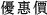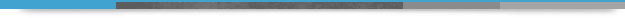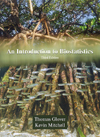## An Introduction to Biostatistics 3/e

+作者：

### Glover

+年份：
2016 年3 版
+ISBN：
9781478627791
+書號：
PS0437PT
+規格：
16開/平裝/單色
+頁數：
560
+出版商：
Waveland Press, Inc

\$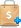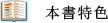For over a decade, Glover and Mitchell have provided life-sciences students with an accessible, complete introduction to the use of statistics in their disciplines. The authors emphasize the relationships between probability, probability distributions, and hypothesis testing using both parametric and nonparametric analyses. Copious examples throughout the text apply concepts and theories to real questions faced by researchers in biology, environmental science, biochemistry, and health sciences. Dozens of examples and problems are new to the Third Edition, as are “Concept Checks”—short questions that allow readers to immediately gauge their mastery of the topics presented. Regardless of mathematical background, all readers will appreciate the value of statistics as a fundamental quantitative skill for the life sciences.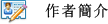Thomas Glover, Kevin Mitchell
For over a decade, Glover and Mitchell have provided life-sciences students with an accessible, complete introduction to the use of statistics in their disciplines. The authors emphasize the relationships between probability, probability distributions, and hypothesis testing using both parametric and nonparametric analyses. Copious examples throughout the text apply concepts and theories to real questions faced by researchers in biology, environmental science, biochemistry, and health sciences. Dozens of examples and problems are new to the Third Edition, as are “Concept Checks”—short questions that allow readers to immediately gauge their mastery of the topics presented. Regardless of mathematical background, all readers will appreciate the value of statistics as a fundamental quantitative skill for the life sciences.1. Introduction to Data Analysis
2. Introduction to Probability
3. Probability Distributions
4. Sampling Distributions
5. Introduction to Hypothesis Testing
6. One-Sample Tests of Hypothesis
7. Tests of Hypothesis Involving Two Samples
8. k-Sample Tests of Hypothesis: The Analysis of Variance
9. Two-Factor Analysis
10. Linear Regression and Correlation
11. Goodness of Fit Tests
Appendix A: Proofs of Selected Results
Appendix B: Answers to Even-Numbered Problems
Appendix C: Tables of Distributions and Critical Values